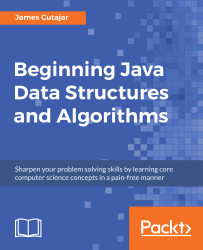•#### Beginning Java Data Structures and Algorithms#### Overview of this book

Learning about data structures and algorithms gives you a better insight on how to solve common programming problems. Most of the problems faced everyday by programmers have been solved, tried, and tested. By knowing how these solutions work, you can ensure that you choose the right tool when you face these problems. This book teaches you tools that you can use to build efficient applications. It starts with an introduction to algorithms and big O notation, later explains bubble, merge, quicksort, and other popular programming patterns. You’ll also learn about data structures such as binary trees, hash tables, and graphs. The book progresses to advanced concepts, such as algorithm design paradigms and graph theory. By the end of the book, you will know how to correctly implement common algorithms and data structures within your applications.
Title PagePackt UpsellContributorsPrefaceFree Chapter
Algorithms and ComplexitiesSorting Algorithms and Fundamental Data StructuresHash Tables and Binary Search TreesString Matching AlgorithmsGraphs, Prime Numbers, and Complexity ClassesOther Books You May EnjoyIndex## Chapter 2. Sorting Algorithms and Fundamental Data Structures

In the previous chapter, we saw how the intersection problem can be improved by using a sorting algorithm. This is common with many problems. If the data is organized in an ordered manner, a more efficient algorithm can be developed. In this chapter, we will start by exploring three types of sorting techniques, which are bubble, quick, and merge sorting. Later, we will learn different ways to organize data using fundamental data structures.

By the end of this chapter, you will be able to:

• Describe how bubble sorting works
• Implement faster sorting with quick sort
• Characterize merge sorting
• Build a linked list data structure
• Implement queues
• Describe the stack data structure